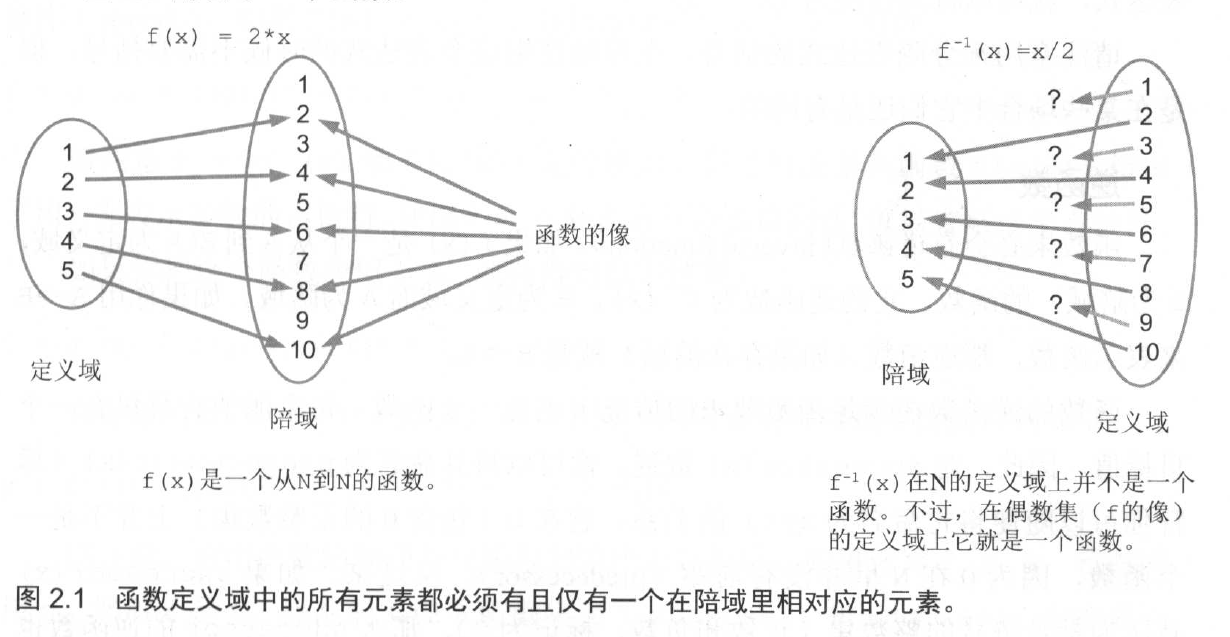# 本章要点

• 理解现实世界中的函数

• 在 Java 中表示函数

• 使用lambda

• 使用高阶函数

• 使用柯里化函数

• 用函数式接口编程

## 学习目标

ps: 虽然有些哲学，但是结果就是如此。这个结果被我们做了缓存，省去了计算的过程

# 现实世界里的函数

ps: 这一段是一些数学定义和概念，你也可以跳过。

## 如何让两个集之间的关系成为函数• 定义域里不存在在陪域里没有对应值的元素。

• 定义域里的一个元素在陪域里不会有两个对应的元素。

• 陪域里的元素可能在源集里没有相对应的元素。

• 陪域里的元素可能在源集里有多个相对应的元素。

• 在定义域里存在对应元素的陪域元素集被称为函数的像（ image of the function ）。

### 例子

f(x) = x + 1


successor(x) = x + 1


predecessor(x) = x + 1


## 逆函数

f (x) = ( 2 * x)


## 复合函数

f · g (5 = f(g(5)) = f(5*2) = (5 * 2 ) + 2


9 。f (5) = g(f(5)) = g(5 + 2) = 7 * 2 = 14


## 多参函数

f(x , y) = x + y

N x N 是所有可能的整数对的集。这个集的元素就是一对整数，更通用的元组（Tuple）表示多个元素的组合，而一对整数其实就是元组的一个特例。

f((3,5)) = 3 + 5 = 8

f(3, 5) = 3+5 = 8

## 函数柯里化

f(x) = g

g(y) = x + y

f(3)(5) = g(5) = 3 + 5 = 8

# 偏应用函数

## 例子

f (rate , price) = price / 100 * ( 100 + rate)

g (price, rate) = price / 100 * (100 + rate)

f (rate) (price )

g (price) (rate)

f(rate）是接收一个价格并返回一个价格的函数。

ps: 个人理解就是可以将变量的一份封装为一个固定的函数，这样便于后续的计算。

《java 函数式编程》